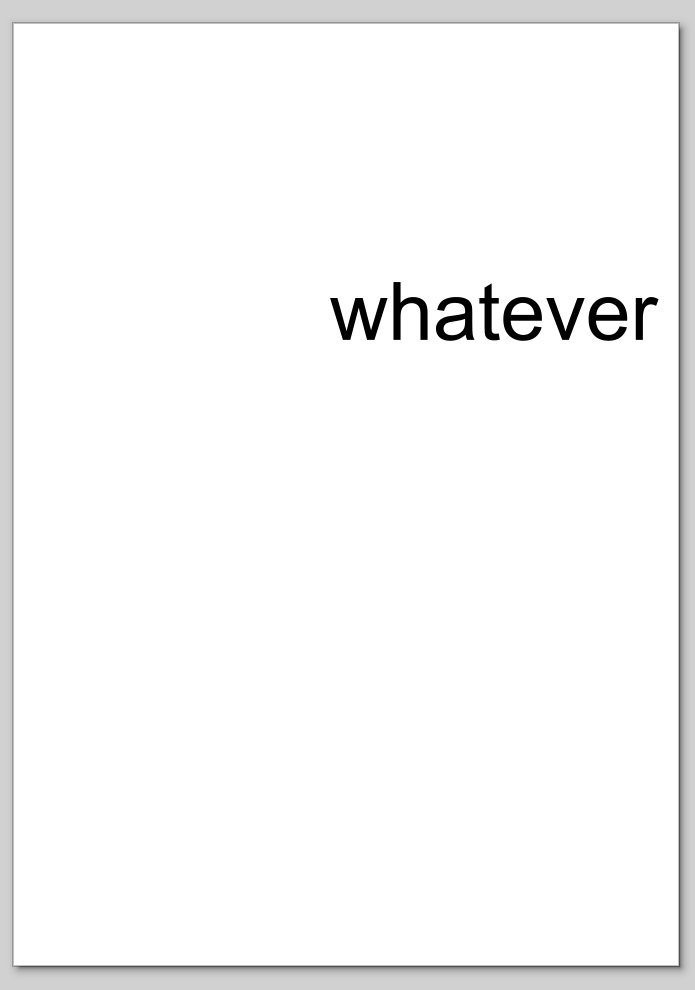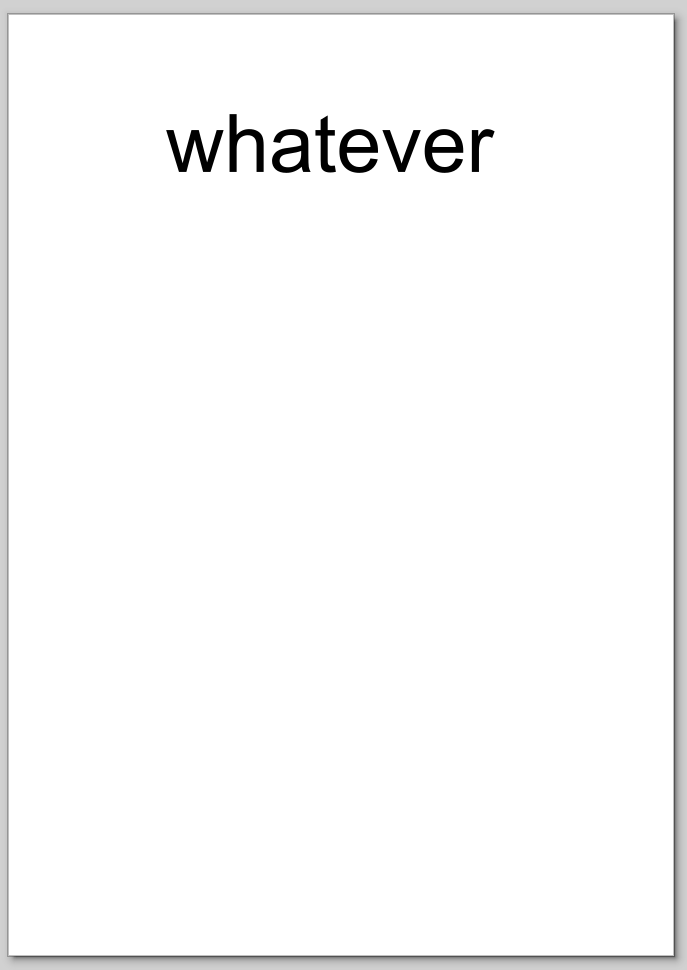TL;DR

Some notes about SVG viewBox and influence on px.

I already imposed upon readers my more-than-fair share of ramblings about SVG, but it’s a big mine and every now and then I hit some topics that require some attention and can use some note-taking.

This time I was playing with putting text in specific places, figuring out how to set the font size.

I’m used to set the font size in pt, i.e. Helvetica 12 or DejaVu Sans Mono for Powerline Book 11. Looking at a stripped-down version generated using InkScape, setting the font size to 72:

<?xml version="1.0">
<svg width="210mm" height="297mm" viewBox="0 0 210 297">
<text style="font-size:25.4px;font-family:Helvetica;stroke-width:0.2"
x="100" y="100">whatever</text>
</svg>


So, the bottom line is that what InkScape shows as 72, is actually saved as 25.4px in the saved file. Unfortunately, forcing 72pt inside the file does not yield the expected result, so let’s look at the maths.

To make a long story short, the px used in the style attribute is the same as the unit used for pretty much everything else, like x and y. Which, in turn, are set according to the viewBox attribute of the upper svg.

In the specific example, the whole x dimension is divided into 210 slots, each representing a px. In the specific case, the actual width is also 210mm, so a px is the same as 1 millimiter.

The above fragment gives us the following:To double check this, we can double the amount of slots in both dimensions, double the font-size, and leave everything else as-is:

<?xml version="1.0">
<svg width="210mm" height="297mm" viewBox="0 0 420 594">
<text style="font-size:50.8px;font-family:Helvetica;stroke-width:0.2"
x="100" y="100">whatever</text>
</svg>


This gives us this:The two strings have the same size, which confirms our hypothesis. They are placed differently, though, because we left the x and y attributes set to 100 as in the previous case, which means that they only go halfway with the new viewBox.

Just before we delve into some maths, it’s useful to observe that the first two values in the viewBox are an offset and do not contribute to the calculation of the size of each unit:

<?xml version="1.0">
<svg width="210mm" height="297mm" viewBox="50 50 210 297">
<text style="font-size:25.4px;font-family:Helvetica;stroke-width:0.2"
x="100" y="100">whatever</text>
</svg>Same size for the string, only a different position (which happens to be the same as in the second example). Whew!

OK, on with the maths. Units in pt are referred to inches; in particular:

$1 [\mathbf{pt}] \equiv \frac{1}{72} [\mathbf{in}]$

In our example, units for the font size are in px, which are related to the overall size (i.e. 210mm for the x size in our examples, corresponding to the width of an A4 page) and to how many slots we want to cut it (i.e. 210 in the viewBox or our first example). This means:

$1 [\mathbf{px}] \equiv \frac{210}{210} [\mathbf{mm}] = 1 [\mathbf{mm}]$

We know that $1 [\mathbf{in}] \equiv 25.4 [\mathbf{mm}]$, so:

$1 [\mathbf{pt}] \equiv \frac{1}{72} [\mathbf{in}] \equiv \frac{25.4}{72} [\mathbf{mm}] \equiv \frac{25.4}{72} [\mathbf{px}]$

This is consistent with what we saw: setting 72 (pt) in InkScape landed us on 25.4px inside the first example.

Let’s double check with the second example, where the viewBox has double the slots across the same actual width:

$1 [\mathbf{pt}] \equiv \frac{1}{72} [\mathbf{in}] \\ 1[\mathbf{px}] \equiv \frac{210}{420} [\mathbf{mm}] = \frac{1}{2} [\mathbf{mm}] \\ 1 [\mathbf{pt}] \equiv \frac{1}{72} [\mathbf{in}] \equiv \frac{25.4}{72} [\mathbf{mm}] \equiv 2 \frac{25.4}{72} [\mathbf{px}] \equiv \frac{50.8}{72} [\mathbf{px}]$

Again, this confirms our observations in the second SVG file, yay!

To make it a bit more general, let’s define some quantities:

• $U$ is the unit used to describe the width and height of the page in the SVG file;
• $C_U$ is the unit conversion factor for $1 [\mathbf{in}]$, i.e. how many units $[\mathbf{U}]$ correspond to $1 [\mathbf{in}]$ (in our example, it would be $25.4$). Its dimensions are $[\mathbf{U} \cdot \mathbf{in}^{-1}]$;
• $W_U$ is the width of the page in the SVG file, in units $[\mathbf{U}]$;
• $S_W$ is the number of slots (i.e. $[\mathbf{px}]$) in the width x direction.

We have:

$1 [\mathbf{pt}] \equiv \frac{1}{72} [\mathbf{in}] \equiv \frac{C_U}{72} [\mathbf{U}] \equiv \frac{C_U \cdot S_W}{72 \cdot W_U} [\mathbf{px}]$

As a double check, in our second example we have:

$1 [\mathbf{U}] \equiv 1 [\mathbf{mm}] \\ C_U = 25.4 [\mathbf{mm} \cdot \mathbf{in}^{-1}] \\ W_U = 210 [\mathbf{mm}] \\ S_W = 420 [\mathbf{px}] \\ 1 [\mathbf{pt}] \equiv \frac{25.4 \cdot 420}{72 \cdot 210} [\mathbf{px}] \equiv \frac{50.8}{72} [\mathbf{px}]$

like we already calculated and checked.

Stay safe!

Comments? Octodon, , GitHub, Reddit, or drop me a line!